Conditional Probability - Statement

Chapter 13 Class 12 Probability
Concept wise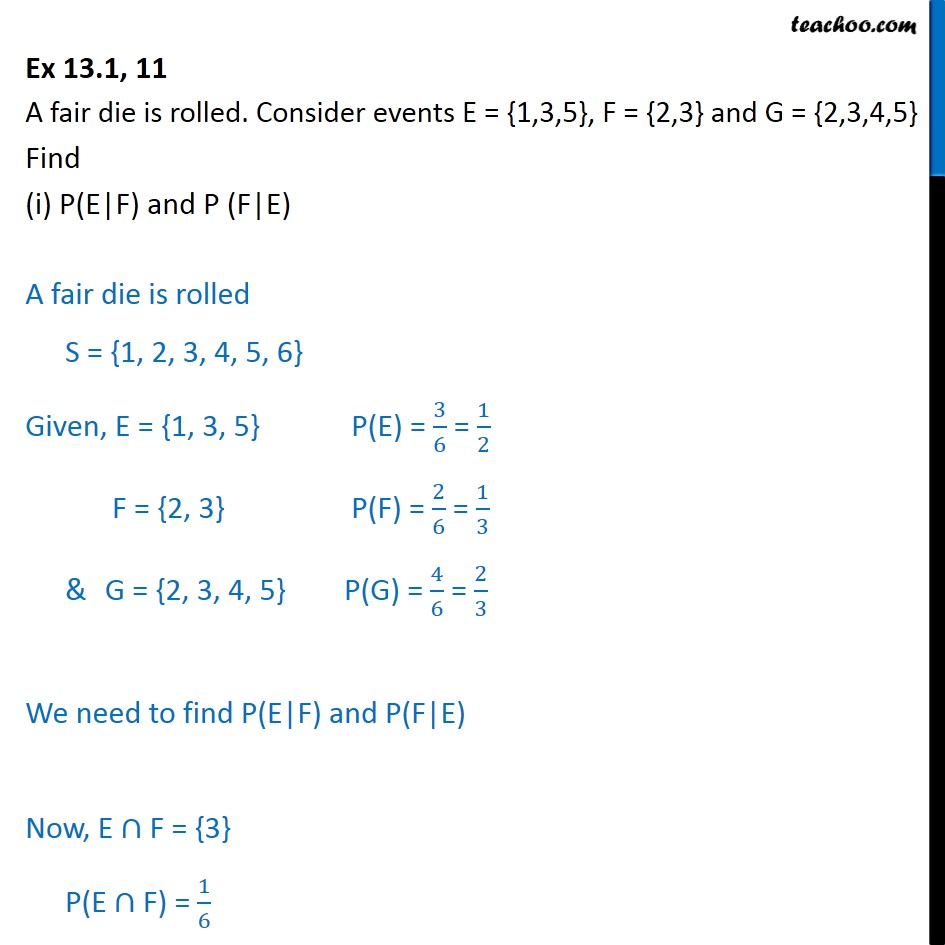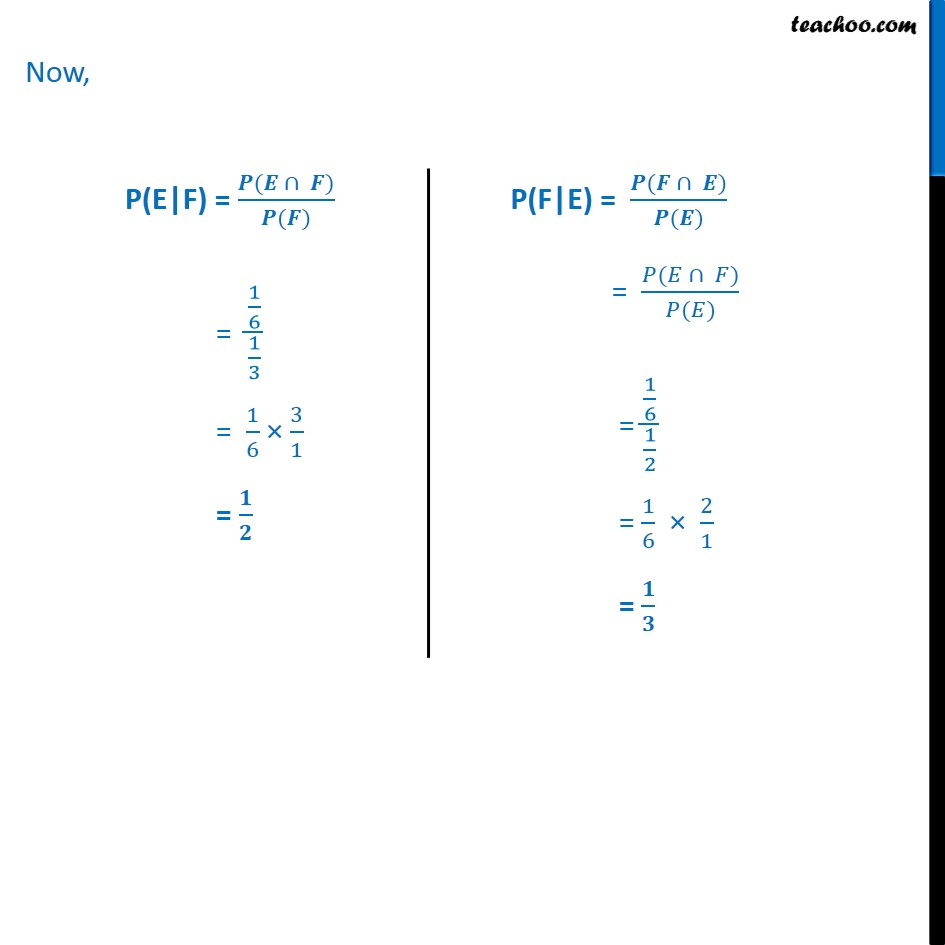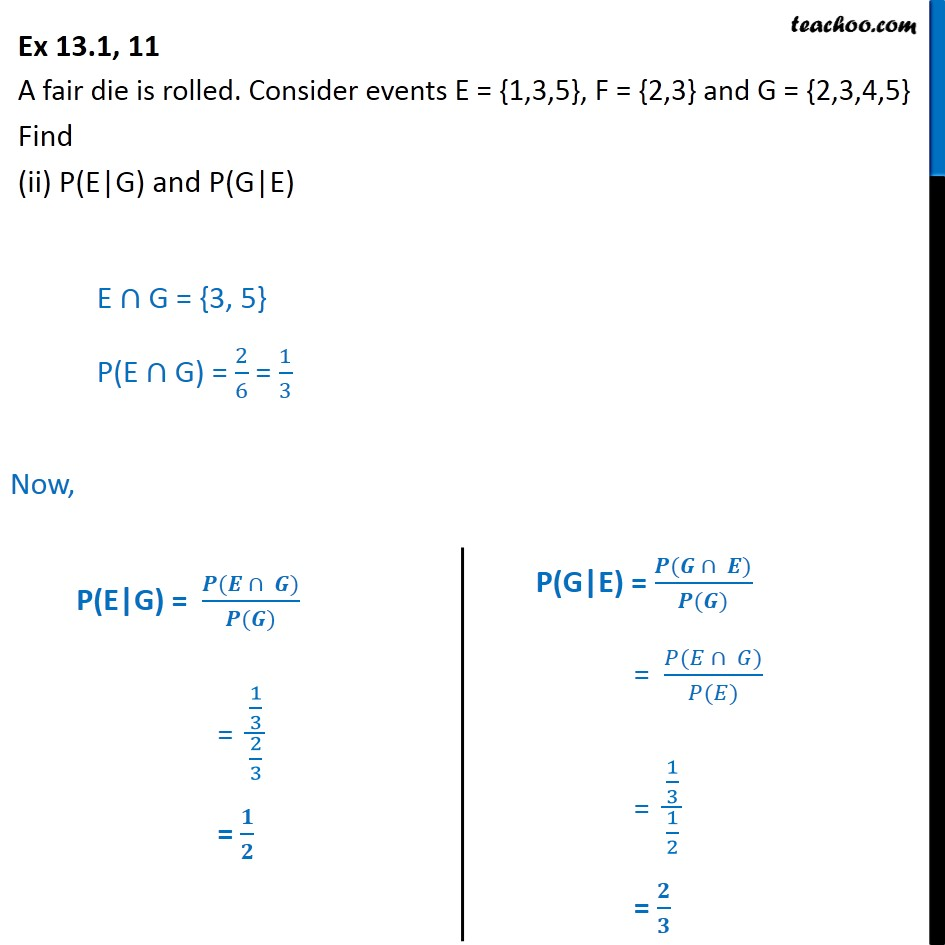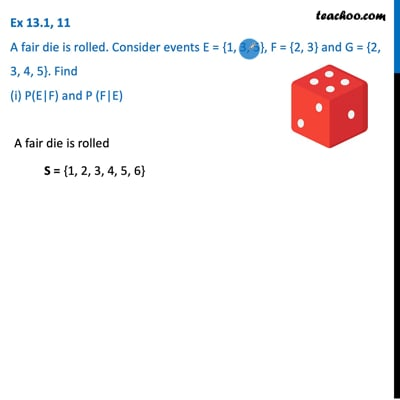This video is only available for Teachoo black users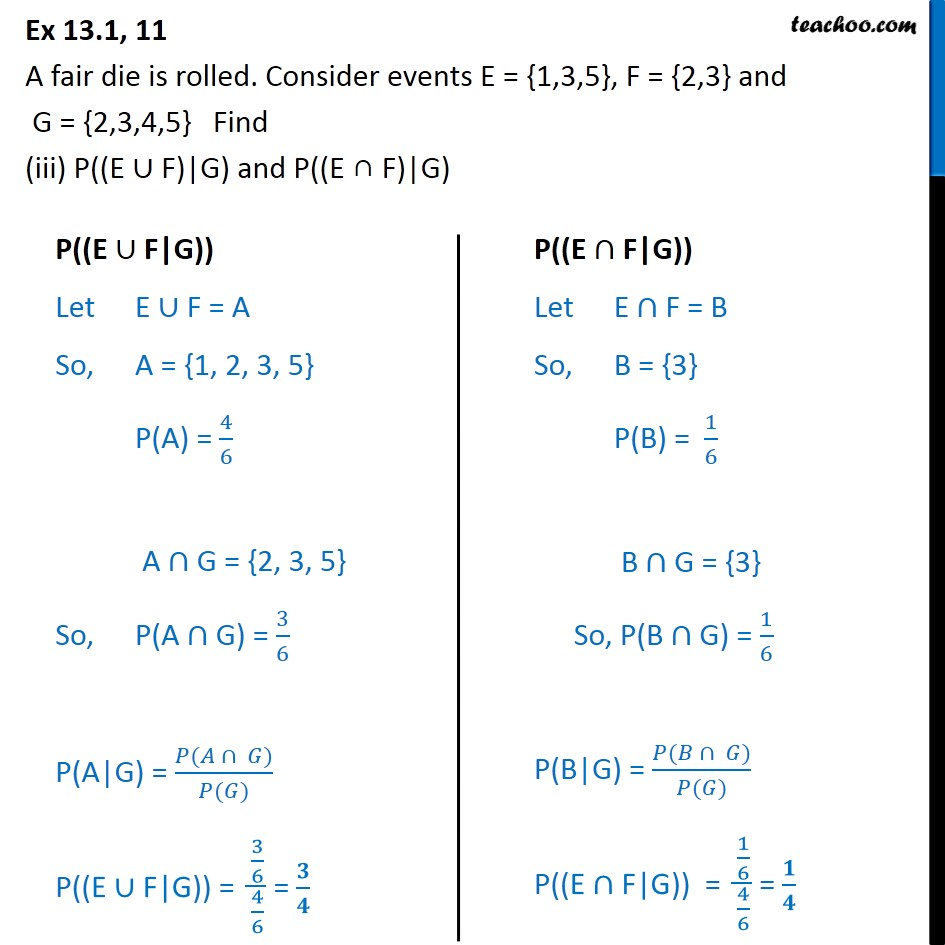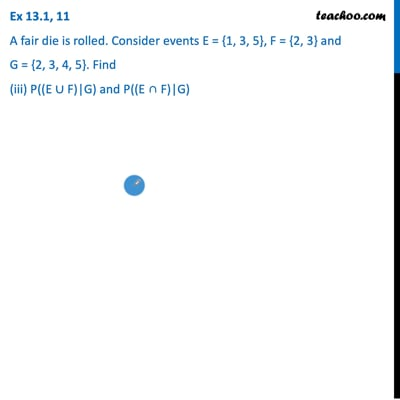This video is only available for Teachoo black users

Introducing your new favourite teacher - Teachoo Black, at only ₹83 per month

### Transcript

Ex 13.1, 11 A fair die is rolled. Consider events E = {1,3,5}, F = {2,3} and G = {2,3,4,5} Find (i) P(E|F) and P (F|E) A fair die is rolled S = {1, 2, 3, 4, 5, 6} Given, E = {1, 3, 5} P(E) = 3﷮6﷯ = 1﷮2﷯ F = {2, 3} P(F) = 2﷮6﷯ = 1﷮3﷯ & G = {2, 3, 4, 5} P(G) = 4﷮6﷯ = 2﷮3﷯ We need to find P(E|F) and P(F|E) Now, E∩F = {3} P(E∩F) = 1﷮6﷯ Now, Ex 13.1, 11 A fair die is rolled. Consider events E = {1,3,5}, F = {2,3} and G = {2,3,4,5} Find (ii) P(E|G) and P(G|E) E∩G = {3, 5} P(E∩G) = 2﷮6﷯ = 1﷮3﷯ Now, Ex 13.1, 11 A fair die is rolled. Consider events E = {1,3,5}, F = {2,3} and G = {2,3,4,5} Find (iii) P((E ∪ F)|G) and P((E ∩ F)|G)